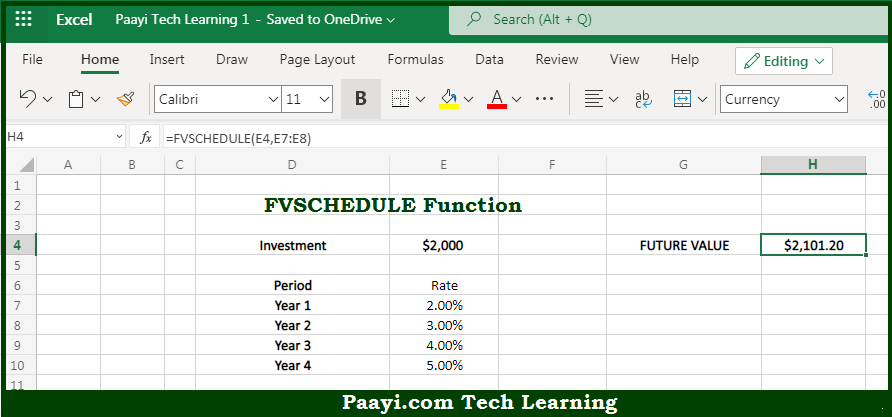# Learn How to Use Microsoft Excel FVSCHEDULE Function

Written by | 0 Comments | 623 Views

In this article, you will learn how to use the Microsoft Excel FVSCHEDULE function and its prime function in Microsoft Excel. You will also get to know the Microsoft Excel FVSCHEDULE function return value and syntax with the help of some examples.

Microsoft Excel FVSCHEDULE Function

The main purpose of the Microsoft Excel FVSCHEDULE function is to get the future value of principal compound interest. That implies, with the help of the FVSCHEDULE function you can able to return the future value of a single sum based on a schedule of given interest rates. It should be noted that the FVSCHEDULE function can be used to find the future value of an investment with a variable or adjustable rate. So, with the help of the FVSCHEDULE function, you can able to get the future value of principal compound interest.

Return Value of FVSCHEDULE Function

The return value will be the future value.

Syntax of FVSCHEDULE Function

=FVSCHEDULE(principal, schedule)

Where the arguments:

• principal: This is the initial investment sum.
• schedule: This is the schedule of interest rates, provided as range or array.

## How to Use Microsoft Excel FVSCHEDULE Function?So we know that Microsoft Excel FVSCHEDULE function you can able to get the future value of principal compound interest. That implies, with the help of the FVSCHEDULE function you can able to return the future value of a single sum based on a schedule of given interest rates. It should be noted that the FVSCHEDULE function can be used to find the future value of an investment with a variable or adjustable rate. So, with the help of the FVSCHEDULE function, you can able to get the future value of principal compound interest.